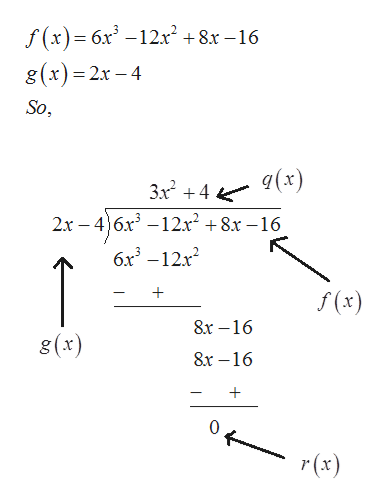# Use polynomial long division to rewrite f as f = g · q + r for each of the following:(a) Divide f(x) = 6x3 − 12x2 + 8x − 16 by the linear polynomial g(x) = 2x − 4.(b) Dividef(x)=3x3 −8x2 +x−5 by the linear polynomial g(x)=3x−2.

Question
1 views

Use polynomial long division to rewrite f as f = g · q + r for each of the following:

(a) Divide f(x) = 6x3 − 12x2 + 8x − 16 by the linear polynomial g(x) = 2x − 4.
(b) Dividef(x)=3x3 −8x2 +x−5 by the linear polynomial g(x)=3x−2.

check_circle

Step 1

Given:

Step 2

Solve (a) by long division:

Here,help_outlineImage Transcriptionclosef(x)= 6x3 -12x2 +8x -16 g(x) 2x -4 So, (x) 3x24 2x 46x12x2 +8x -16 6x3 -12x2 1 f(x) 8х —16 g(x) 8х -16 0 r(x) fullscreen
Step 3

Now, substitute the value of f(...

### Want to see the full answer?

See Solution

#### Want to see this answer and more?

Solutions are written by subject experts who are available 24/7. Questions are typically answered within 1 hour.*

See Solution
*Response times may vary by subject and question.
Tagged in

### Other